# Beta distribution

Use the beta distribution for random variables between 0 and 1. The beta distribution is frequently used to model the distribution of order statistics—for example, the kth order statistic from a sample of n uniform (0, 1) variables has a beta (k, n + 1 – k) distribution—and to model events that are defined by minimum and maximum values. The beta distribution is often rescaled to model the time to completion of a task. The beta distribution is also used in Bayesian statistics, for example, as the prior distribution of a binomial probability.

The beta distribution is a continuous distribution defined by two shape parameters. The distribution can take on different shapes depending on the values of the two parameters.

Both shapes equal 1

When both shapes equal 1, the beta distribution is the uniform distribution.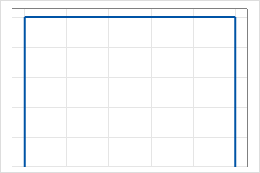Both shapes are less than 1

When both shapes are less than 1, the distribution is U-shaped.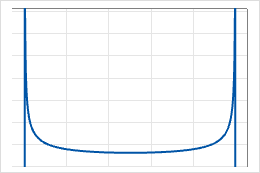Both shapes are the same and are greater than 1

When both shapes are equal and greater than 1, the distribution is symmetric.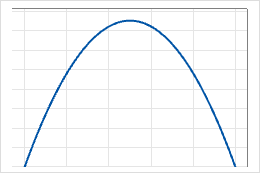First shape is greater than second shape

When the first shape is greater than the second shape, the distribution is skewed to the left.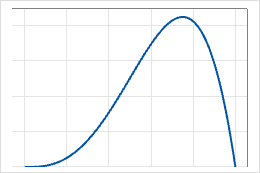First shape is less than second shape

When the first shape is less than second shape, the distribution is skewed to the right.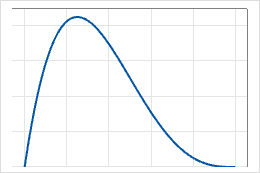By using this site you agree to the use of cookies for analytics and personalized content.  Read our policy